## Jones Polynomial

The second Knot Polynomial discovered. Unlike the first-discovered Alexander Polynomial, the Jones polynomial can sometimes distinguish handedness (as can its more powerful generalization, the HOMFLY Polynomial). Jones polynomials are Laurent Polynomials in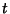assigned to an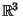Knot. The Jones polynomials are denoted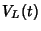for Links,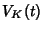for Knots, and normalized so that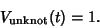(1)

For example, the Jones polynomial of the Trefoil Knot is given by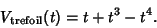(2)

If a Link has an Odd number of components, then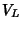is a Laurent Polynomial over the Integers; if the number of components is Even,is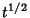times a Laurent Polynomial. The Jones polynomial of a Knot Sumsatisfies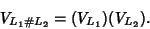(3)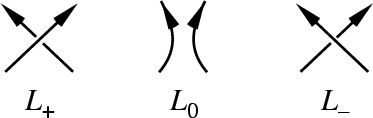The Skein Relationship for under- and overcrossings is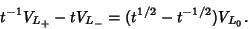(4)

Combined with the link sum relationship, this allows Jones polynomials to be built up from simple knots and links to more complicated ones.

Some interesting identities from Jones (1985) follow. For any Link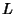,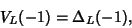(5)

where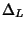is the Alexander Polynomial, and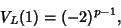(6)

where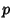is the number of components of. For any Knot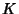,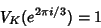(7)

and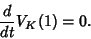(8)

Let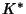denote the Mirror Image of a Knot. Then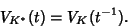(9)

For example, the right-hand and left-hand Trefoil Knots have polynomials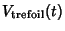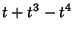(10)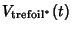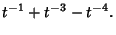(11)

Jones defined a simplified trace invariant for knots by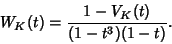(12)

The Arf Invariant of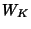is given by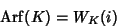(13)

(Jones 1985), where i is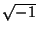. A table of the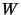polynomials is given by Jones (1985) for knots of up to eight crossings, and by Jones (1987) for knots of up to 10 crossings. (Note that in these papers, an additional polynomial which Jones calls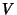is also tabulated, but it is not the conventionally defined Jones polynomial.)

Jones polynomials were subsequently generalized to the two-variable HOMFLY Polynomials, the relationship being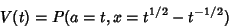(14)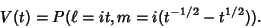(15)

They are related to the Kauffman Polynomial F by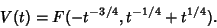(16)

Jones (1987) gives a table of Braid Words andpolynomials for knots up to 10 crossings. Jones polynomials for Knots up to nine crossings are given in Adams (1994) and for oriented links up to nine crossings by Doll and Hoste (1991). All Prime Knots with 10 or fewer crossings have distinct Jones polynomials. It is not known if there is a nontrivial knot with Jones polynomial 1. The Jones polynomial of an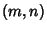-Torus Knot is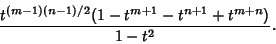(17)

Let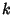be one component of an oriented Link. Now form a new oriented Link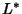by reversing the orientation of. Then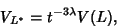(18)

whereis the Jones polynomial and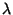is the Linking Number ofand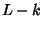. No such result is known for HOMFLY Polynomials (Lickorish and Millett 1988).

Birman and Lin (1993) showed that substituting the Power Series for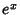as the variable in the Jones polynomial yields a Power Series whose Coefficients are Vassiliev Polynomials.

Letbe an oriented connected Link projection ofcrossings, then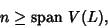(19)

with equality ifis Alternating and has no Removable Crossing (Lickorish and Millett 1988).

There exist distinct Knots with the same Jones polynomial. Examples include (05-001, 10-132), (08-008, 10-129), (08-016, 10-156), (10-025, 10-056), (10-022, 10-035), (10-041, 10-094), (10-043, 10-091), (10-059, 10-106), (10-060, 10-083), (10-071, 10-104), (10-073, 10-086), (10-081, 10-109), and (10-137, 10-155) (Jones 1987). Incidentally, the first four of these also have the same HOMFLY Polynomial.

Witten (1989) gave a heuristic definition in terms of a topological quantum field theory, and Sawin (1996) showed that the quantum group''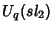gives rise to the Jones polynomial.

References

Adams, C. C. The Knot Book: An Elementary Introduction to the Mathematical Theory of Knots. New York: W. H. Freeman, 1994.

Birman, J. S. and Lin, X.-S. Knot Polynomials and Vassiliev's Invariants.'' Invent. Math. 111, 225-270, 1993.

Doll, H. and Hoste, J. A Tabulation of Oriented Links.'' Math. Comput. 57, 747-761, 1991.

Jones, V. A Polynomial Invariant for Knots via von Neumann Algebras.'' Bull. Am. Math. Soc. 12, 103-111, 1985.

Jones, V. Hecke Algebra Representations of Braid Groups and Link Polynomials.'' Ann. Math. 126, 335-388, 1987.

Lickorish, W. B. R. and Millett, B. R. The New Polynomial Invariants of Knots and Links.'' Math. Mag. 61, 1-23, 1988.

Murasugi, K. Jones Polynomials and Classical Conjectures in Knot Theory.'' Topology 26, 297-307, 1987.

Praslov, V. V. and Sossinsky, A. B. Knots, Links, Braids and 3-Manifolds: An Introduction to the New Invariants in Low-Dimensional Topology. Providence, RI: Amer. Math. Soc., 1996.

Sawin, S. Links, Quantum Groups, and TQFTS.'' Bull. Amer. Math. Soc. 33, 413-445, 1996.

Stoimenow, A. Jones Polynomials.'' http://www.informatik.hu-berlin.de/~stoimeno/ptab/j10.html.

Thistlethwaite, M. A Spanning Tree Expansion for the Jones Polynomial.'' Topology 26, 297-309, 1987.Weisstein, E. W. Knots and Links.'' Mathematica notebook Knots.m.

Witten, E. Quantum Field Theory and the Jones Polynomial.'' Comm. Math. Phys. 121, 351-399, 1989.